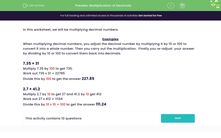# Multiply Decimals

In this worksheet, students will multiply decimal numbers.Key stage:  KS 3

Curriculum topic:   Number

Curriculum subtopic:   Use Four Operations for All Numbers

Popular topics:   Decimals worksheets

Difficulty level:#### Worksheet Overview

In this activity, we will be multiplying decimal numbers.

The easiest way to do this is to remove the decimal point from the numbers, multiply them together and then put the decimal point back in again.

We need to count how many numbers there are after the decimal point and make sure that there are the same number after the decimal point in the answer.

For example:

7.35 × 31

There are two numbers after the decimal point.

Remove the decimal point and do the calculation 735 x 31

735 x 31 = 22785

Finally, put the decimal point back in. There must be the same number of decimal places in the answer as there were in the original calculation, so that is 2.

So, 7.35 x 31 = 227.85

2.7 × 0.412

Count the numbers after the decimal point. There are 4.

Remove the decimal point and do the calculation 27 x 412

412 x 27 = 11124

Finally, add the decimal point back in: 1.1124

2.7 x 0.412 = 1.1124

Let's have a go at some questions now.

### What is EdPlace?

We're your National Curriculum aligned online education content provider helping each child succeed in English, maths and science from year 1 to GCSE. With an EdPlace account you’ll be able to track and measure progress, helping each child achieve their best. We build confidence and attainment by personalising each child’s learning at a level that suits them.

Get started